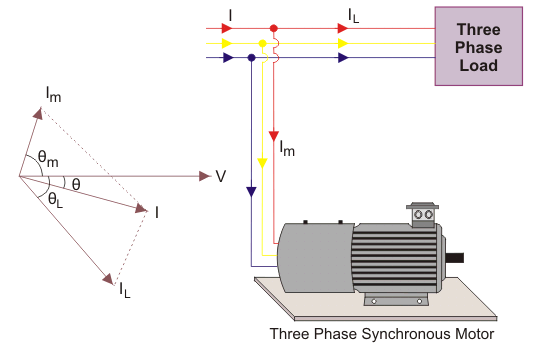# Synchronous Condenser

Like capacitor bank, we can use an overexcited synchronous motor to improve the poor power factor of a power system. The main advantage of using synchronous motor is that the improvement of power factor is smooth.
When a synchronous motor runs with over-excitation, it draws leading current from the source. We use this property of a synchronous motor for the purpose.

Here, in a three-phase system, we connect one three-phase synchronous motor and run it at no load.Suppose due to a reactive load of the power system the system draws a current IL from the source at a lagging angle θL in respect of voltage. Now the motor draws a IM from the same source at a leading angle θM. Now the total current drawn from the source is the vector sum of the load current IL and motor current IM. The resultant current I drawn from the source has an angle θ in respect of voltage. The angle θ is less than angle θL. Hence power factor of the system cosθ is now more than the power factor cosθL of the system before we connect the synchronous condenser to the system.

The synchronous condenser is the more advanced technique of improving power factor than a static capacitor bank, but power factor improvement by synchronous condenser below 500 kVAR is not economical than that by a static capacitor bank. For major power network we use synchronous condensers for the purpose, but for comparatively lower rated systems we usually employ capacitor bank.

The advantages of a synchronous condenser are that we can control the power factor of system smoothly without stepping as per requirement. In case of a static capacitor bank, this fine adjustments of power factor cannot be possible rather a capacitor bank improves the power factor stepwise.
The short circuit withstand-limit of the armature winding of a synchronous motor is high.

Although, synchronous condenser system has some disadvantages. The system is not silent since the synchronous motor has to rotate continuously.